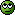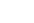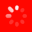The No.1 Website for Pro Audio
All  This Thread  Reviews  Gear Database  Gear for saleLatest  Trending
Lives for gear

I believe Soundman2020 posted the equations in the topic New construction 2-ch listening room build questions. Look for where he writes, "Here's the full set of equations for roughly estimating the isolation in each of the three frequency ranges."
Lives for gear

You bratme to it Starlight. Thank you.
Lives for gear

Quote:
Please post the equations! It would be most appreciated! Thanks!
Sure! No problem. It's actually a set of equations that you need for estimating overall isolation of a two-leaf wall in the various regions of the spectrum. All of the equations are fairly simple:

First, for a single-leaf barrier you need the Mass Law equation:

TL = 14.5 log (M * 0.205) + 23 dB

Where: M = Surface density in kg/m2

For the complete two-leaf wall, you need to calculate the above for EACH leaf separately (call the results "R1" and "R2").

Then you need to know the resonant frequency of the system, using the MSM resonance equation:

f0 = C [ (m1 + m2) / (m1 x m2 x d)]^0.5

Where:
C=constant (60 if the cavity is empty, 43 if you fill it with suitable insulation)
m1=mass of first leaf (kg/m^2 or lbs/ft2)
m2 mass of second leaf (kg/m^2 or lbs/ft2)
d=depth of cavity (m or ft)

(NOTE: C=60 for imperial empty cavity, 2650 metric empty cavity, 43 for imperial full, 1900 for metric full)

Then you use the following three equations to determine the isolation that your wall will provide for each of the three frequency ranges:

R = 20log(f * (m1 + m2) ) - 47 ...[for the region where f < f0]
R = (R1 + R2)/2 + 20log(f * d) - 29 ...[for the region where f0 < f < f1]
R = R1 + R2 + 6 ...[for the region where f > f1]

Where:
m1 and m2 are still the surface densities of leaf 1 and leaf 2, respectively
f0 is the resonant frequency from the MSM resonant equation,
f1 is 55/d Hz
R1 and R2 are the transmission loss numbers you calculated first, using the mass law equation for each leaf

And that's it! Nothing complex. High school math. It's just simple addition, subtraction, multiplication, division, square roots, and logarithms.

For a three-leaf wall (such as when you have an extra layer of drywall, MLV, or anything else in the middle of the wall), its rather more complex, but the outcome is always, without exception, that the isolation for such a 3-leaf wall will be worse than for the equivalent 2-leaf wall, all other factors being equal, and regardless of the materials used. The issue is the mass of each leaf, and the size of the air gaps. There will be variations, of course, from some materials, but the OVERALL result is that the total isolation will be worse in low frequencies, probably better in the mid range, and about the same or maybe better in high frequencies.

- Stuart -
Lives for gear

Quote:
I believe Soundman2020 posted the equations in the topic New construction 2-ch listening room build questions. Look for where he writes, "Here's the full set of equations for roughly estimating the isolation in each of the three frequency ranges."
And both you and Andre beat me to it! I didn't check for new posts before I posted mine, above ...Sorry about that!

Oh well, I guess it does no harm to post twice...- Stuart -
Lives for gearSoundman2020, Starlight, and avare thanks for posting! The space that I’m renting has some “challenges” that I have to overcome, this is useful information!
Lives for gear

Orville redenbocker makes \$5 every time anyone leaves a comment on gearslutz. Anyone who plays their stock need only follow GS forums closely.

Welcome to the Gearslutz Pro Audio Community!

Registration benefits include:
• The ability to reply to and create new discussions
• Access to members-only giveaways & competitions
• Interact with VIP industry experts in our guest Q&As
• Access to members-only sub forum discussions
• Get INSTANT ACCESS to the world's best private pro audio Classifieds for only USD \$20/year
• Promote your eBay auctions and Reverb.com listings for free
• Remove this message!
You need an account to post a reply. Create a username and password below and an account will be created and your post entered.